Courses

# Ketones, Biomolecules, Chemistry In Action, P-Block

## 25 Questions MCQ Test Mock Test Series for JEE Main & Advanced 2022 | Ketones, Biomolecules, Chemistry In Action, P-Block

Description
This mock test of Ketones, Biomolecules, Chemistry In Action, P-Block for JEE helps you for every JEE entrance exam. This contains 25 Multiple Choice Questions for JEE Ketones, Biomolecules, Chemistry In Action, P-Block (mcq) to study with solutions a complete question bank. The solved questions answers in this Ketones, Biomolecules, Chemistry In Action, P-Block quiz give you a good mix of easy questions and tough questions. JEE students definitely take this Ketones, Biomolecules, Chemistry In Action, P-Block exercise for a better result in the exam. You can find other Ketones, Biomolecules, Chemistry In Action, P-Block extra questions, long questions & short questions for JEE on EduRev as well by searching above.
QUESTION: 1

Solution:
QUESTION: 2

Solution:
QUESTION: 3

### Concentrated nitric acid is a

Solution:
QUESTION: 4
Which of the following oxoacids of sulphur contains an S–S bond ?
Solution:
QUESTION: 5
Which of the following acids is used in lead storage batteries as an electrolyte ?
Solution:
QUESTION: 6
Which of the following bonds has the highest energy ?
Solution:
QUESTION: 7
Conectrated HCl contains
Solution:
QUESTION: 8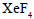reacts violently with water to give
Solution:
QUESTION: 9
Which of the following compounds on heating at about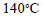gives acetic acid ?
Solution:
QUESTION: 10
Consider the following compounds with regard to their acidities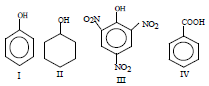The correct order of decreasing acidity of these compounds is
Solution:
QUESTION: 11
The compound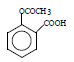is a/an
Solution:
QUESTION: 12
The following reactions are carried out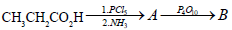The end product (B) is
Solution:
QUESTION: 13
Which of the following is a chiral molecule ?
Solution:
QUESTION: 14
Consider the following sequence of reactions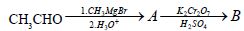The product (B) is
Solution:
QUESTION: 15
The reagent that can distinguish benzaldehyde from propionaldehyde is
Solution:
QUESTION: 16

Consider the following sequence of reactionsThe final product (B) is

Solution:
QUESTION: 17
Consider the following reactions of the compound (X)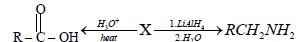The compound (X) is expected to be
Solution:
QUESTION: 18
Consider the following compounds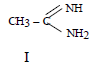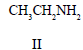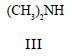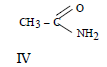The correct order of basicity of the above compounds is
Solution:
QUESTION: 19
(+ )-Sucrose is made up of
Solution:
QUESTION: 20
Mutarotation involves
Solution:
*Answer can only contain numeric values
QUESTION: 21

A detergent (C12H25SO-4Na+) solution becomes a colloidal solution at 10–3 M. On an average 1013 colloidal particals are present in 1mm3. What is the average number of ions are contain in one colloidal particles (NA = 6 × 1023)

Solution:

No. of sodium lauryl sulphate in 1ℓ solution
= 10–3 × 6 × 1023
= 6 × 1020
No. of compound per mm3  = 6× 1014
No. of colloidal particles per mm3  = 1013
No. of molecular per colloidal particles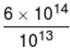= 60

*Answer can only contain numeric values
QUESTION: 22

When mixture of NaCl and K2Cr2O7 is gently warmed with conc. H2SO4, then compound 'X' of Cr is formed. Oxidation state of Cr in 'X' = a; Number of non-axial d-orbitals in hybridisation of Cr in 'X' = b
Find, (a + b)

Solution: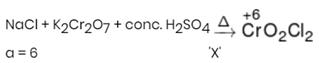Hybridisation Cr in 'X' is d3s. (In d3s, non-axial d-orbital, dxy, dyz and dxz participate)
b = 3
a + b = 9

*Answer can only contain numeric values
QUESTION: 23

How many amide isomers exist for C4H9ON that do not form amine on treatment with Br2-NaOH ?

Solution:

2° and 3° amine isomer donot form amine on treatment with Br2 + NaOH.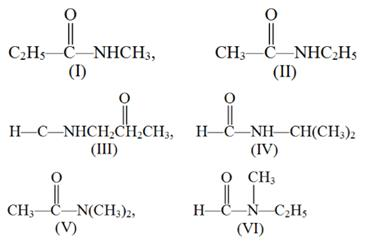*Answer can only contain numeric values
QUESTION: 24

How many carbanion is more stable than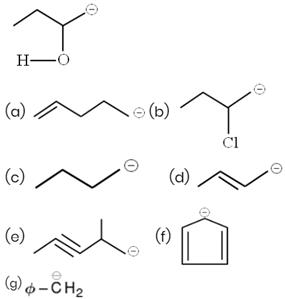Solution:
*Answer can only contain numeric values
QUESTION: 25

How many carbocation is less stable than allylcarbocation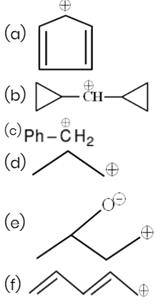Solution: